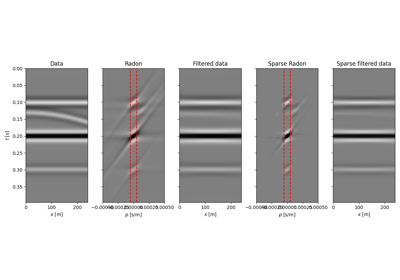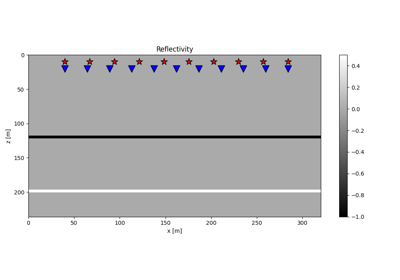# pylops.optimization.sparsity.FISTA¶

pylops.optimization.sparsity.FISTA(Op, data, niter, eps=0.1, alpha=None, eigsiter=None, eigstol=0, tol=1e-10, returninfo=False, show=False, threshkind='soft', perc=None, callback=None, decay=None, SOp=None)[source]

Fast Iterative Shrinkage-Thresholding Algorithm (FISTA).

Solve an optimization problem with $$L_p, \; p=0, 1/2, 1$$ regularization, given the operator Op and data y. The operator can be real or complex, and should ideally be either square $$N=M$$ or underdetermined $$N<M$$.

Parameters: Op : pylops.LinearOperator Operator to invert data : numpy.ndarray Data niter : int Number of iterations eps : float, optional Sparsity damping alpha : float, optional Step size ($$\alpha \le 1/\lambda_{max}(\mathbf{Op}^H\mathbf{Op})$$ guarantees convergence. If None, the maximum eigenvalue is estimated and the optimal step size is chosen. If provided, the condition will not be checked internally). eigsiter : int, optional Number of iterations for eigenvalue estimation if alpha=None eigstol : float, optional Tolerance for eigenvalue estimation if alpha=None tol : float, optional Tolerance. Stop iterations if difference between inverted model at subsequent iterations is smaller than tol returninfo : bool, optional Return info of FISTA solver show : bool, optional Display iterations log threshkind : str, optional Kind of thresholding (‘hard’, ‘soft’, ‘half’, ‘soft-percentile’, or ‘half-percentile’ - ‘soft’ used as default) perc : float, optional Percentile, as percentage of values to be kept by thresholding (to be provided when thresholding is soft-percentile or half-percentile) callback : callable, optional Function with signature (callback(x)) to call after each iteration where x is the current model vector decay : numpy.ndarray, optional Decay factor to be applied to thresholding during iterations SOp : pylops.LinearOperator, optional Regularization operator (use when solving the analysis problem) xinv : numpy.ndarray Inverted model niter : int Number of effective iterations cost : numpy.ndarray, optional History of cost function NotImplementedError If threshkind is different from hard, soft, half, soft-percentile, or half-percentile ValueError If perc=None when threshkind is soft-percentile or half-percentile

OMP
Orthogonal Matching Pursuit (OMP).
ISTA
Iterative Shrinkage-Thresholding Algorithm (ISTA).
SPGL1
Spectral Projected-Gradient for L1 norm (SPGL1).
SplitBregman
Split Bregman for mixed L2-L1 norms.

Notes

Solves the following synthesis problem for the operator $$\mathbf{Op}$$ and the data $$\mathbf{d}$$:

$J = ||\mathbf{d} - \mathbf{Op} \mathbf{x}||_2^2 + \epsilon ||\mathbf{x}||_p$

or the analysis problem:

$J = ||\mathbf{d} - \mathbf{Op} \mathbf{x}||_2^2 + \epsilon ||\mathbf{SOp}^H\mathbf{x}||_p$

if SOp is provided.

The Fast Iterative Shrinkage-Thresholding Algorithm (FISTA)  is used, where $$p=0, 1, 1/2$$. This is a modified version of ISTA solver with improved convergence properties and limited additional computational cost. Similarly to the ISTA solver, the choice of the thresholding algorithm to apply at every iteration is based on the choice of $$p$$.

  Beck, A., and Teboulle, M., “A Fast Iterative Shrinkage-Thresholding Algorithm for Linear Inverse Problems”, SIAM Journal on Imaging Sciences, vol. 2, pp. 183-202. 2009.

## Examples using pylops.optimization.sparsity.FISTA¶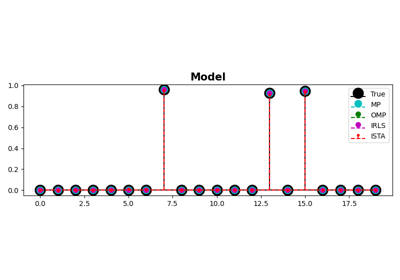MP, OMP, ISTA and FISTA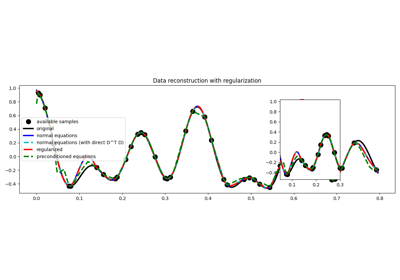03. Solvers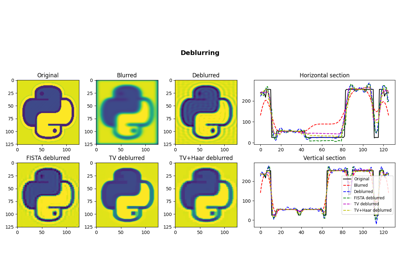05. Image deblurring